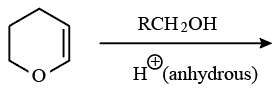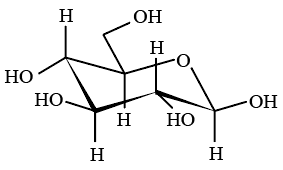Instructions

For the following questions answer them individually

Question 1

# Oxidation states of the metal in the minerals haematite and magnetite, respectively, areQuestion 2

# Among the following complexes $$(K-P), K_3[Fe(CN)_6](K), [Co(NH_3)_6]Cl_3 (L), Na_3[Co(oxalate)_3] (M), [Ni(H_2O)_6]Cl_2 (N), K_2[Pt(CN)_4] (O)$$ and $$[Zn(H_2O)_6](NO_3)_2 (P)$$ the diamegnetic complexes areQuestion 3

# Passing $$H_2S$$ gasinto a mixture of $$Mn^{2+}, Ni^{2+}, Cu^{2+}$$ and $$Hg^{2+}$$ ions in an acidified aqueous solution precipitatesQuestion 4

# Consider the following cell reaction:$$2Fe_{(s)} + O_{2(g)} + 4H^+_{(aq)} \rightarrow 2Fe^{2+}_{(aq)} + 2H_2O(l) E^\circ = 1.67 V$$ At $$[Fe^{2+}] = 10^{-3} M, P(O_2) = 0.1$$ atm and pH = 3, the cell potential at $$25^\circ C$$ isQuestion 5

# The freezing point (in $$^\circ C$$) of a solution containing 0.1 g of $$K_3[Fe(CN)_6](Mol. Wt. 329)$$ in 100 g of water $$(K_f = 1.86 K kg mol^{-1})$$ isQuestion 6

# Amongst the compounds given, the one that would form a brilliant colored dye on treatment with $$NaNO_2$$ in dil. HCl followed by addition to an alkaline solution of $$\beta$$-naphthol isQuestion 7

# The major product of the following reaction isQuestion 8

# The following carbohydrate isQuestion 9

# Reduction of the metal centre in aqueous permanganateion involvesQuestion 10

# The equilibrium$$2Cu^\mid \rightleftharpoons Cu^O + Cu^\parallel$$in aqueous medium at $$25 ^\circ C$$ shifts towards the left in the presence ofOR